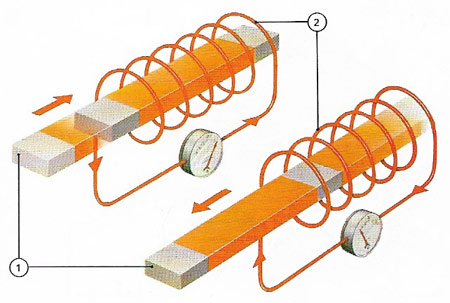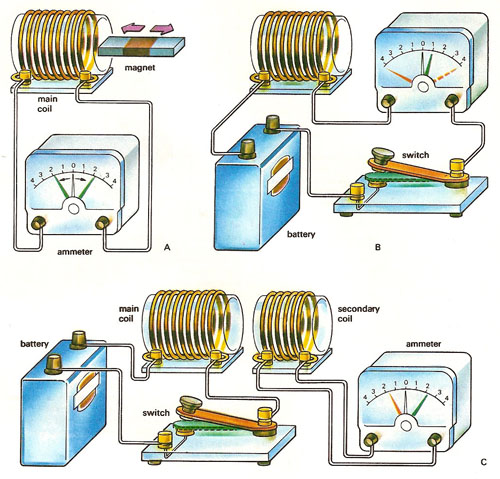A# electromagnetic inductionElectricity can be generated by a magnet, an electrical conductor, and relative movement. Moving the magnet (1) causes the flux lines surrounding it to cut the conductor of the coil (2) and induce in it a voltage, coinciding with the movement. The faster the movemnt, the higher the voltage induced. Opposite movements produce opposite voltages – any current in a circuit between the coil ends flows first one way then the other as the magnet is moved in and out. These alternating currents and the generators are called alternators.Simple experiments in electromagnetic induction. (A) When a permanent magnet is being moved in and out of a small coil, and "induced" current flows in the coil. But it is only while the magnetic field in the coil is changing that any induced current flows. (B) Self-induction occurs when a circuit contains an inductive component such as a coil. When a circuit is completed through a coil (by closing a switch), the increasing current in the coil gives rise to a changing magnetic field which interacts with the coil itself, setting up an electromotive force which opposes the original current. A sensitive ammeter in series with such a coil might show a momentary current (red) in the direction opposite to the subsequent steady current (green). On breaking the circuit, a similar forward current surge (broken red) occurs. (C) Mutual induction is exhibited when there is a magnetic linkage between two circuits containing inductive elements. Closing the switch in the primary circuit creates a magnetic field in the secondary circuit, giving rise to a momentary current as in (A). Opening the switch in the primary causes an equivalent current in the reverse direction in the secondary.

Electromagnetic induction is the phenomenon in which an electric field is generated in an electric circuit when the number of magnetic field lines passing through the circuit changes. Electromagnetic induction was discovered independently by Michael Faraday and Joseph Henry. The voltage induced is proportional to the rate of change of the field, and large voltages can be produced by switching off quite small magnetic fields suddenly. Frequently, the magnetic field is itself generated by an electric current in a coil, in which case the voltage induced is proportional to the rate of change of the current (see inductance).

The principle finds numerous applications in electric generators and motors, transformers, microphones, and engine ignition systems. In the less familiar technique of induction heating, widely used in metal working, an object is heated by currents created in it by the voltage induced by a high-frequency current in a nearby coil; as the coil field will pass through insulators without heating them, the principle can be applied to produce "cold hob" electric stoves.

## Lenz's law

This law of electromagnetic induction, deduced in 1834 by the Russian physicist Heinrich Lenz (1804–1865), states that the electromotive force (emf) induced in a circuit is such as to oppose the flux change giving rise to it.